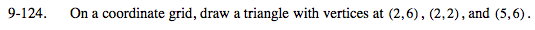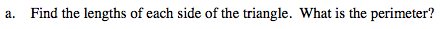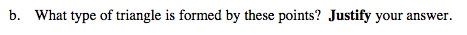### Home > MC2 > Chapter 9 > Lesson 9.3.4 > Problem9-124

9-124.To find the hypotenuse, we can use the Pythagorean theorem: a2 + b2 = c2
If the legs are 3 and 4, how long is the hypotenuse?
After finding the hypotenuse, find the perimeter.The triangle has a right angle so it is a right triangle.